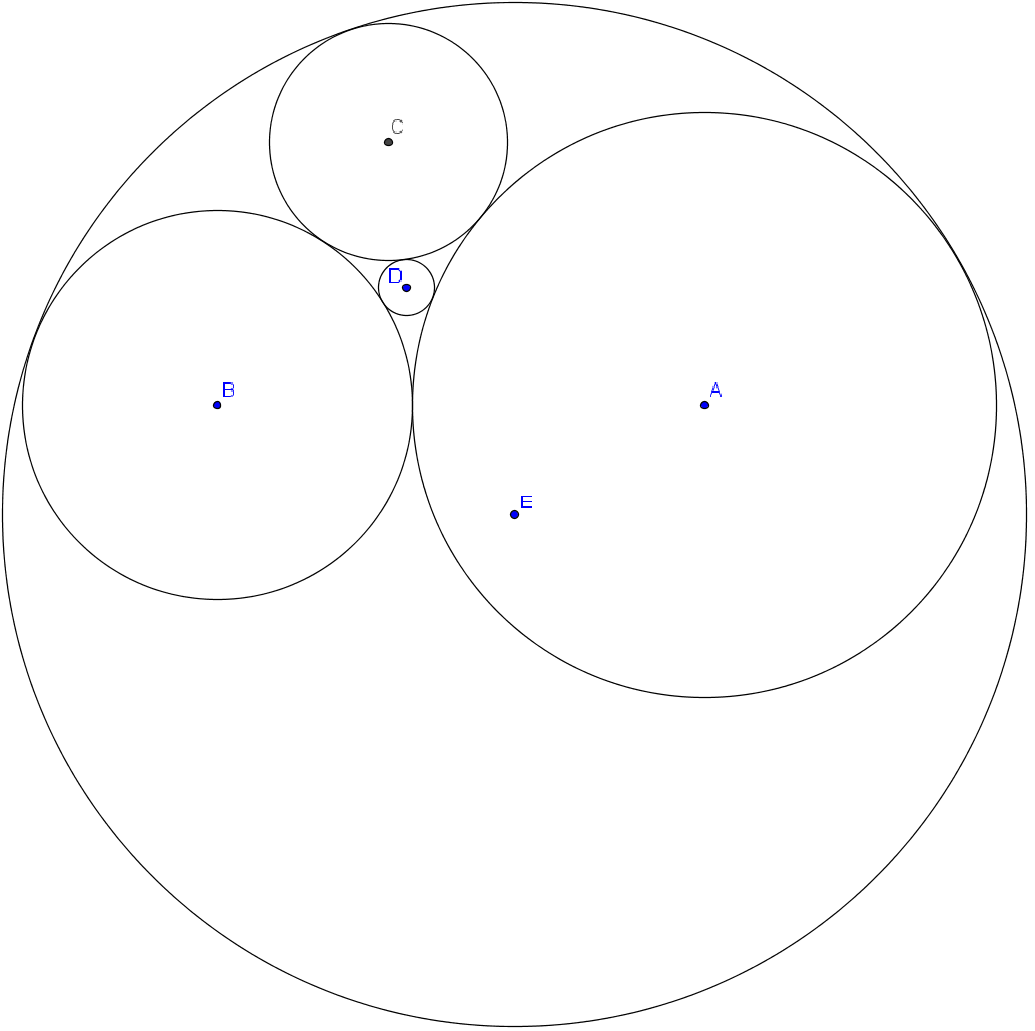# Crazy circles

Geometry Level 5Three circles mutually tangent with centers $A$, $B$ and $C$ have radii of $21$, $14$ and $c$ respectively. Let the circle with center $D$ and radius $2$ be externally tangent to the three circles, and let the circle with center $E$ and radius $R$ be internally tangent to the three circles. Find $\lfloor 100(c+R) \rfloor$.

×